Lu, W., Hu, Y., Yao, J., and Li, Y. (2013). "Optimization and simulation research of tensile properties of wood lap joint," BioRes. 8(1), 1409-1419.

#### Abstract

The performance of veneer joints is known to affect the quality of laminated veneer lumber (LVL), so experimental research and simulation analysis of the tensile properties of lap joints were performed and reported in this paper. The lap length, specimen thickness, and specimen width were selected as the experimental factors. The maximum tensile load increased with the increase of each factor; the tensile strength increased with the increase of lap length, whereas it decreased with the increase of specimen thickness. Specimen width had significant effect on the maximum tensile load, but had little influence on the tensile strength. A response surface model of tensile strength was obtained using Matlab software, and it was used to predict the tensile properties for lap joints. The results of ANSYS simulation analysis showed that the stress peaks were concentrated in the joint ends; the peak shear stress and peak stripping stress all decreased with the increase of lap length and increased with the increase of specimen thickness; the result was consistent with the experimental results; therefore, the finite element simulation results can be used for the optimized selection of size parameters of joints.

Optimization and Simulation Research of Tensile Properties of Wood Lap Joint

Wei Lu,a,b Yingcheng Hu,a,* Jia Yao,a,b and Yanfang Li a

The performance of veneer joints is known to affect the quality of laminated veneer lumber (LVL), so experimental research and simulation analysis of the tensile properties of lap joints were performed and reported in this paper. The lap length, specimen thickness, and specimen width were selected as the experimental factors. The maximum tensile load increased with the increase of each factor; the tensile strength increased with the increase of lap length, whereas it decreased with the increase of specimen thickness. Specimen width had significant effect on the maximum tensile load, but had little influence on the tensile strength. A response surface model of tensile strength was obtained using Matlab software, and it was used to predict the tensile properties for lap joints. The results of ANSYS simulation analysis showed that the stress peaks were concentrated in the joint ends; the peak shear stress and peak stripping stress all decreased with the increase of lap length and increased with the increase of specimen thickness; the result was consistent with the experimental results; therefore, the finite element simulation results can be used for the optimized selection of size parameters of joints.

Keywords: Lap joint; Tensile performance; Finite element simulation; Response surface model

Contact information: a: Key Laboratory of Bio-based Material Science and Technology of Ministry of Education of China, College of Material Science and Engineering, Northeast Forestry University, Harbin,150040, China; b: College of Mechanical Engineering, Jiamusi University, 154007, Jiamusi, China. * Corresponding author: yingchenghu@163.com

INTRODUCTION

Laminated veneer lumber (LVL) is a useful type of wooden structural material that must meet the requirements of actual use, particularly regarding mechanical strength (Li et al. 2011; Zhang and Chui 1994). Because of the limits of the production process, veneer must undergo longitudinal lengthening, and veneer joints are inevitably produced. The longitudinal extension can adopt three joint forms: butt joint, scarf joint, and lap joint (Chen and Dai 2000; Tang et al. 2006). The scarf joint has the best mechanical performance, but the manufacturing processes of lap joints and butt joints are relatively simple, so the lap joint is selected as the research object in this study. The joints are the weakest links in the LVL structure; therefore, researching joints performance is of great significance (Lu et al. 2012).

Up to the present, researchers have reported applications of lap joints for metal and composite materials (Chen et al. 2009; Li et al. 2006; Tao et al. 2008). In some studies the performance of wood joints has also been reported. By using the Volkersen’s shear lag model (Gindl et al. 2012), highly significant effects of adhesive stiffness were found at short overlap length and high specimen thickness. The bond strength of scarf joint and lap, as well as the joint testing method for spruce and beech wood glued with different adhesives has been researched (Konnerth et al. 2006). The failure mode in standard lap joint tests was mainly wood failure, whereas scarf joints failed in the bond line. The shear-strength-predicting capabilities of test specimens for wood-adhesive bonds have been studied (Serrano 2004), and the results showed that the prediction of bond line strength was highly dependent on both the specimen type used and adhesive properties such as strength, fracture energy, and the shape of the stress-slip behavior of the adhesive layer.

With the wide application of LVL, the lap joint as the main lap method has received more attention, but the joint stress change analysis of different structure size has not been studies in detail (Dong and Wang 2010; Hirofumi et al. 2010; Souza et al. 2010). Moreover, many studies have focused on the influence of tensile performance on the joints with the constant size of different adhesive or different tree species. The present research was conducted with the same adhesive type and tree species, and an analysis of the influence of the structure size change of lap joint on the tensile properties and the stress distribution was carried out. The main factors affecting the tensile properties was obtained through the tensile experiment of the lap joint; the effective prediction of the joint tensile strength was realized through the response surface fitting equation provided by Matlab software; and the reasonable range of the joint size parameters was determined through the finite element simulation. The comprehensive use of the above methods makes it possible to realize the optimization design of tensile properties of lap joint, and can further improve the mechanical performance of longitudinal extension LVL.

EXPERIMENTAL

Materials

Birch (Betula platyphylla Suk) wood was used for this study and was purchased from the Yichun forest region of Heilongjiang, China. Its moisture content was 8 to 10%. Polyvinyl acetate emulsion (PVAC) made in Herbin, China was selected as an adhesive to simplify the bonding process, because it facilitated rapid curing at room temperature. The adhesive emulsion had a solids content of 50%, a viscosity of 5000 to 15,000 MPa•s, and a density of 1.05 g/cm3. European standard “EN 205:2003” was followed to make lap joint specimens, as illustrated in Fig. 1.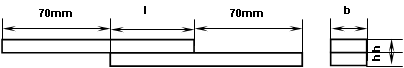Fig. 1. Illustration of lap joints of lap length l, thickness h, and width b

Experimental Design

An orthogonal design of experiments (3 factors 3 levels) was used to study lap joint strength with regards to lap length l, specimen thickness h, and specimen width (Table 1). All experiments were performed on specimens that had been conditioned for a week at 20°C and 65% relative humidity, and each group test contained eight samples.

Table 1. Factors and Levels of ExperimentExperimental Methods

Tensile strength testing was performed with a SANS-CMT5504 universal mechanical testing machine in accordance with the wood-based panel standard GB/T17657-1999 of China and EN 302-1-2004 of Europe. The maximum tensile load (P1max) was used to calculate the tensile strength (Eq. 1) to achieve the tensile strength σt: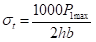(1)

where σt is tensile strength (MPa) and P1max is maximum tensile load (kN). The data from the tensile testing experiments are given in Table 2.

The response surface method is a type of optimization process statistical test design. A continuous variable surface model can be established by using the method. Based on such a model it is then possible to evaluate the impact of different factors as well as their interactions and their optimal levels. Response surfaces have been success-fully applied to various kinds of process optimization. In this study the selected variables were lap length l and specimen thickness h. The predicted variable was tensile strength.

Simulation analysis of the tensile performance of birch single lap joints was carried out using the ANSYS finite element method; ANSYS software was adopted to realize a valid simulation of tensile conditions of wood joints and to reveal the principles governing the tensile forces required for breakage.

Results and analysis

Results and Analysis for Destructive Tensile Test

After the destructive tensile testing, three forms of specimen failure can be observed: adhesive failure, adherend failure (wood failure), and mixed failure. All the failure forms of the specimens were judged to involve mixed failure in the present study. The optimal structural design of a lap joint can be obtained by intuitive analysis of variances (Cheng 2005), as shown in Table 2. Through the comparisons of extreme difference in intuitive analysis, the relative importance of each factor can be determined, and the optimum plan for each mechanical index can be obtained. The order of the impact of each factor on P1max was b > l > h, and the optimum scheme was determined as b3l3h3. As in Table 2, P1max increased with lb, and h increasing, and b had the greatest influence among all the factors. However, the value of b is always constant in applications involving LVLs, so the factor b can be ignored. The order of the impact of each factor on σt was h > l > b. The optimum scheme was determined as h1l3b1 as in Table 2. The value of σt decreased with hincreasing, and increased with l increasing. Because the extreme differences in the value of b were very small, the influence of b could be ignored.

Gu (2003) reported the tensile load is proportional to the specimen width. In other words the quotient P1max / b is constant; therefore, had no effect on the tensile strength as calculated by Equation (1). Factors l and can be regarded as the research factors of the tensile property in the following Matlab response surface analysis and ANSYS finite element simulate analysis.

Table 2. Tensile Experimental Results and Intuitive Analysis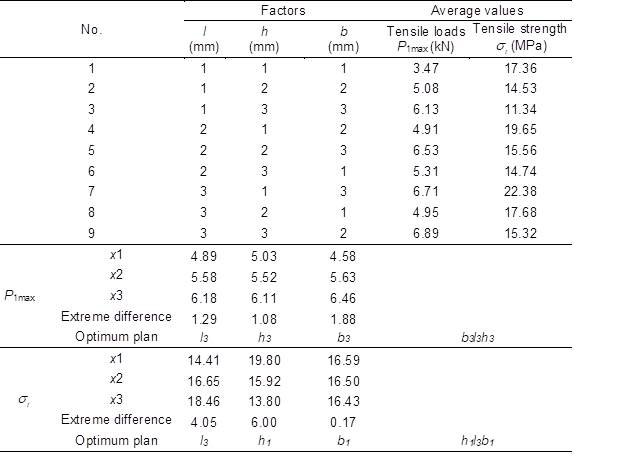Tensile Strength Prediction Model

A continuous variable surface model of tensile strength was established by using the response surface method. Based on the tensile strength values in Table 2, the response surface of the tensile strength was obtained by using Matlab software, and results are shown in Fig. 2. The quantities l and h were set as the independent variables, and the response surface fitting equation can be expressed as Equation (2).=30.5496-0.0005l2+0.1898l+0.2187h2-4.3017h-0.0065lh (2)

The coefficient of determination, R2, for the response surface model was 0.9749, indicating that Equation (2) represented the data well. The predicted values of tensile strength determined by the model are compared to the experimentally measured tensile strength in Table 3. The maximum absolute value of the variation coefficient was 5.64%, which indicates that Equation (2) could predict the tensile strength of lap joints with reasonable precision. The response surface method was thus judged to be suitable for process optimization of the tensile strength of lap joints.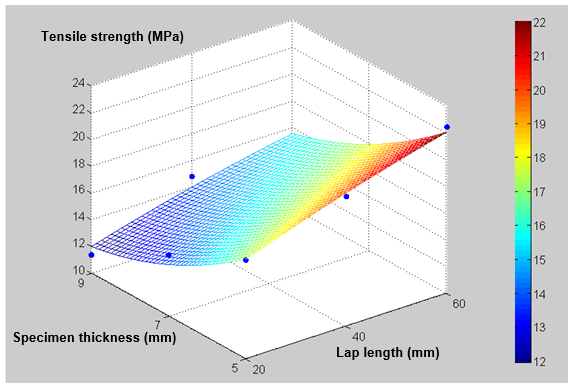Fig. 2. Response surface model predicting the impact of l and on lap joint tensile strength

Table 3. Predicted Values Compared to Measured Tensile Strength and their Variation Coefficient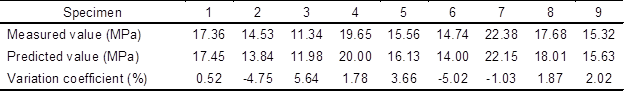Model Building for Finite Element Simulation

Since factor b had a small influence on the tensile strength, this factor could be ignored in order to simplify the simulation analysis. A two-dimensional simulation analysis by using ANSYS software was performed utilizing the factors l and h. The measured physical and mechanical performance of birch is shown in Table 4 (Lu et al. 2011). The constraints and loading conditions of the model simulation of the tensile test were as shown in Fig. 3. The “Quad 8 node 82” unit was adopted as the geometric model. A tensile load “F” was loaded, using a value of 10 MPa. The simulation adopted a linear elastic analysis, and the mesh tool was used to determine the suitable mesh size.

Table 4. Physical and Mechanical Performance of Birch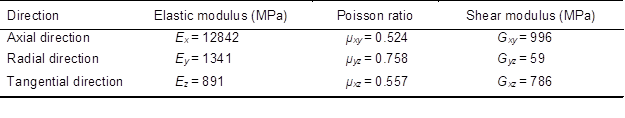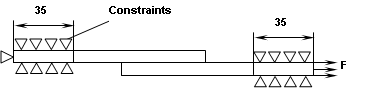Fig. 3. Loading and constraints of the tensile model of lap joints

Finite Element Simulation Analysis

The tensile equivalent stress of lap joints when l = 40 mm and h = 5 mm can be obtained in Fig. 4 through the simulation analysis. The stress values present the point symmetrical distribution, and the maximum stress peaks occur in the joint ends, so the joint ends were determined as the places at which end-cracking and failure phenomena occur, and this finding coincided with the results of the tensile destructive experiment.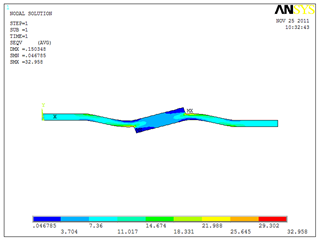Fig. 4. Tensile equivalent stress of lap joints when l =40 mm and h = 5 mm

Under tensile loads a lap joint will bear both a shear stress paralleled to the tensile direction and a stripping stress vertical to the tensile direction. Due to the difference of stress directions, the stripping stress is more likely to lead to joint cracking and finally failure. Figure 5 shows the stress distribution curves of the total shear stress and the stripping stress of birch lap joints for various lap lengths (l = 10 to 60 mm) at a fixed value of 5 mm, and the peak values of shear stress and of stripping stress all occurred at the joint ends. The values of stress peak decreased with increasing lap length, but the decline tendency was less steep. The stress values near the center of the lap length were gradually tending to zero.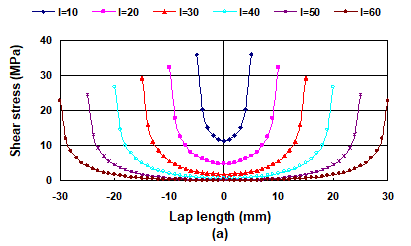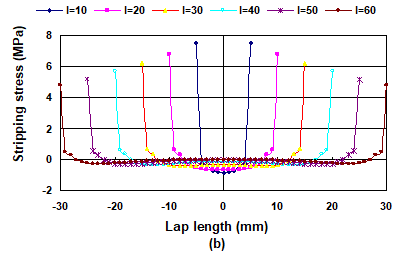Fig. 5. Tensile stress distribution curves for different lap length of lap joints: (a) Shear stress distribution curves when h = 5 mm; (b) Stripping stress distribution curves when = 5 mm

When l = 50 mm, the stress distribution curves of shear stress and stripping stress for different h values (= 5, 7, 9 mm) are shown in Fig. 6 (a) and (b). The peak values of both stresses were found to increase with the increase of h. Figure 7 shows the changing of peak stress. When the h value increased from 5 mm to 7 mm, the peak shear stress increased by 4.66 MPa units (20.86%) and the peak stripping stress increased by 1.71 MPa (32.17%); when hwas increased from 5 mm to 9 mm, the peak shear stress increased by 9.05 MPa (40.46%) and the peak stripping stress increased by 3.32 MPa (62.32%). The increased amplitude of the stripping stress peak was greater than the shear stress with the increase of h. The above changing tendency can be rationalized as follows: When the specimen is very thin, the stripping stress is small, so the failure of a lap joint is primarily caused by the shear stress; with the increase of h, the proportion of stripping stress is increased, so the cracking damage is able to occur more readily. Because the bonding strength is constant for a lap joint (with given lap length and specimen width), the tensile strength will decrease with increasing h.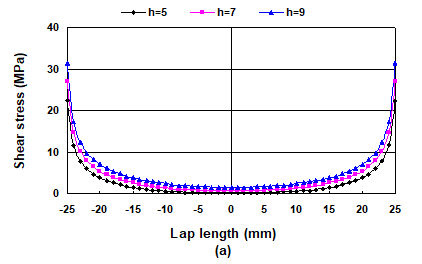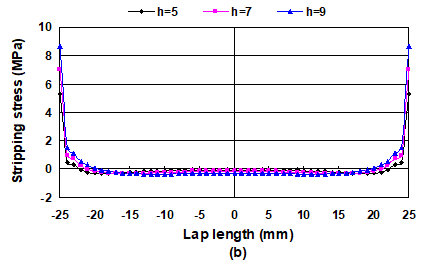Fig. 6. Tensile stress distribution curves of different specimen width of lap joints: (a) Shear stress distribution curves when l = 50 mm; (b) Stripping stress distribution curves when l = 50 mm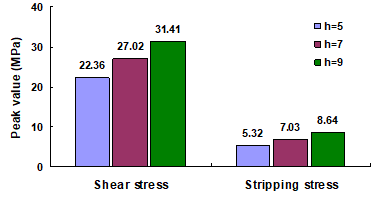Fig. 7. Peak shear and stripping stress for different h values for when l = 50 mm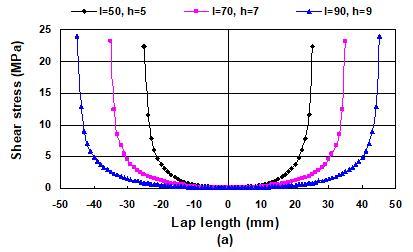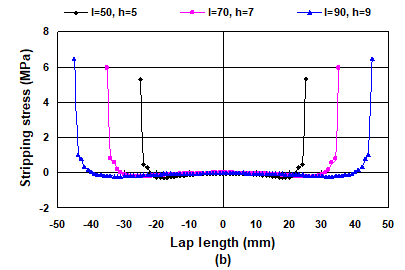Fig. 8. Tensile stress distribution curves of the same length-to-thickness ratio: (a) Shear stress distribution curves when l : h of 10; (b) Stripping stress distribution curves when l : h of 10

From the above simulation analysis, the peak stress decreased with the increase of and increased with the increase of h, which was consistent with the experimental results. Thus, the variables and were found to play a comprehensive role relative to the tensile performanceConsidering the conclusion that the stress distribution was only related to length-to-thickness ratio (Zhang 1986), when setting the length-to-thickness ratio to 10 as an example, adopting three type samples with h=5 mm and l=50 mm; h=7 mm and l=70 mm; h=9 mm and l=90 mm, respectively, the simulation results are as shown in Fig. 8. The middle region stress values were all very near to zero, but the peak stress values were not the same. As in Fig. 9, h increased from h=5 mm and l=50 mm to h=7 mm and l=70 mm, the peak shear stress increased by 0.84 MPa units (3.76%) and the peak stripping stress increased by 0.65 MPa units (12.15%); from h=5 mm and l=50 mm to h=9 mm and l=90 mm, the peak shear stress increased by 1.60 MPa units (7.15%), and the peak stripping stress increased by 1.16 MPa units (21.74%). So the conclusion that the stress distribution was only related to length-to-thickness (Zhang 1986) was not correct. The h value was the most important influencing factor, which was consistent with the experimental analysis.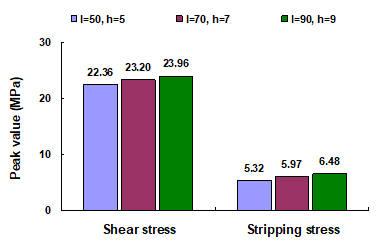Fig. 9. Peak shear and stripping stress for different h and l values for a fixed l : h ratio of 10

CONCLUSIONS

1. By means of comprehensive intuitive statistical analysis, the order of the impact of each size factor on the maximum tensile load was determined as b > l > h. The maximum tensile load increased with the increase of each factor. The order of the impact of the three factors on the tensile strength was h > l > b. The tensile strength decreased with the increase of h, and it increased with the increase of l, whereas the influence of b was minor and could be ignored.

2. The surface response fitting equation of the tensile strength, taking l and h as independent variables, was obtained by using Matlab software. The surface fitting precision was high, and the equation was judged to be suitable for use in the tensile strength prediction calculation of birch lap joint, thus providing a basis for the optimization design of LVL.

3. Finite element simulation analysis of the tensile property of birch lap joint was carried out, and the equivalent stress graph and the stress distribution curves were obtained. The peak stress of lap joint decreased with increasing l, but the decline tendency was less steep; the peak stress was gradually increasing with increasing h, and the increased amplitude of the stripping stress peak was greater than that of the shear stress; the destruction form of the lap joint changed from shear rupture to stripping rupture; the joints were more susceptible to cracking and damage, thus the tensile strength was reduced.

4. Besides the tensile destructive experiments, the surface response method and finite element simulation were adopted to obtain a predictive model and an optimized analysis of tensile performance of wood lap joints. These research methods can make a positive contribution for the optimization of the design of wood lap joints in future applications.

ACKNOWLEDGMENTS

This project was supported by the Fundamental Research Funds for the Central Universities (DL12EB03), National Natural Science Foundation of China (31170516, 31010103905, 51203061).

REFERENCES CITED

Chen, G., and Dai, J. (2000). “The effect of two kinds extension methods on the veneer properties of heavy plywood,” Building Artificial Boards 3, 21-23. (Chinese)

Chen, X., Li, Y., Shi, F., Zhao, H., and Ma, X. (2009). “Influences of adherent thickness, temperature and velocity on strength of adhesively-bonded single-lap joints,” Explosion and Shock Waves 29(5), 449-456. (Chinese)

Cheng, K. (2005). Design and Analysis of Experiments, Tsinghua Univ.Press, Beijing.

Dong, G., and Wang, J. (2010). “Experimental study on the structural performance of poplar laminated veneer lumber beams,” J. Huaiyin Inst. Technol.19(1), 84-88.

Gindl-Altmutter, W., Müller, U., Konnerth, J. (2012). “The significance of lap-shear testing of wood adhesive bonds by means of Volkersen’s shear lag model,” Eur. J. Wood Prod. 70, 903-905.

Gu, J. (2003). Gluing Theory and Gluing Basis, Science Press, Beijing. Chinese.

Hirofumi, I., Hirofumi, N., Hideo, K., Atsushi, M., and Yasushi, H. (2010). “Strength properties of laminated veneer lumber in compression perpendicular to its grain,” J. Wood Sci. 56(5), 422-428.

Li, W., Ma, C., and Shen, S. (2011). “Research progress and prospect on laminated veneer lumber,” Wood Processing Machinery 4, 35-39. (Chinese)

Li, Z., You, M., Zheng, X., and Yu, S. (2006). “The orthogonal test of numerical modeling on the peck stress distributed in lap joint,” China Adhesives15(11), 10-13.

Lu, W., Hu, Y. C., and Yao, J. (2012). “Simulation analysis and nondestructive testing of flexural performance of wood single lap glued joints,” BioResources 7(4), 5391-5400.

Serrano, E. (2004). “A numerical study of the shear-strength-predicting capabilities of test specimens for wood–adhesive bonds,” Int. J. Adhes. Adhes. 24, 23-35.

Souza, F., Menezzi, C., and Júnior, G. (2010). “Material properties and nondestructive evaluation of laminated veneer lumber (LVL) made from Pinus oocarpa and P. kesiya,” Eur. J. Wood Prod. 69(2), 183-192.

Tang, Z., Zhou, S., Hu, S., and Wen, Y. (2006). “Study on slip joint for poplar veneer,” China Wood Industry. 20(6), 23-25. (Chinese)

Tao, Y., Jiao, G., Wang, B., and Chang, Y. (2008). “Effect of the overlap length on the connecting performance of the single-lap joint reinforced by Z-pins,” J. of Materials Sci. and Eng. 26(4), 593-598. (Chinese)

Zhang, F. (1986). “Analysis of adhesive lap joint,” Applied Mathematics and Mechanics 7(10), 877-885. (Chinese)

Zhang, H., and Chui, Y. (1994). “Effects of resin impregnation and process parameters on some properties of poplar LVL,” Forest Prod. J. 44(7/8), 76-78. (Chinese)

Article submitted: Oct. 6, 2012; Peer review completed: Jan. 8, 2012; Revised version received: Jan. 18, 2013; Accepted: Jan. 28, 2013; Published: January 30, 2013.